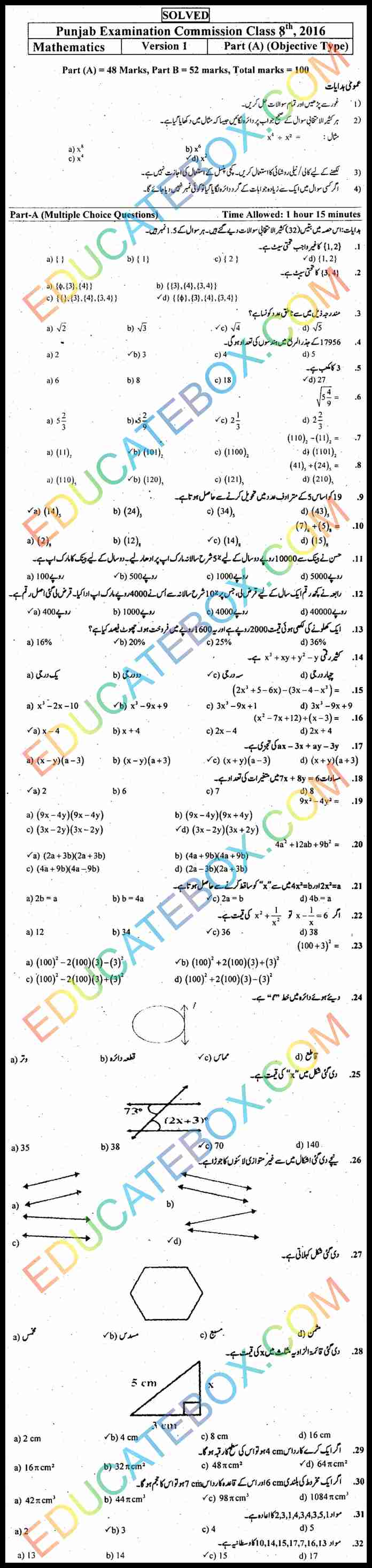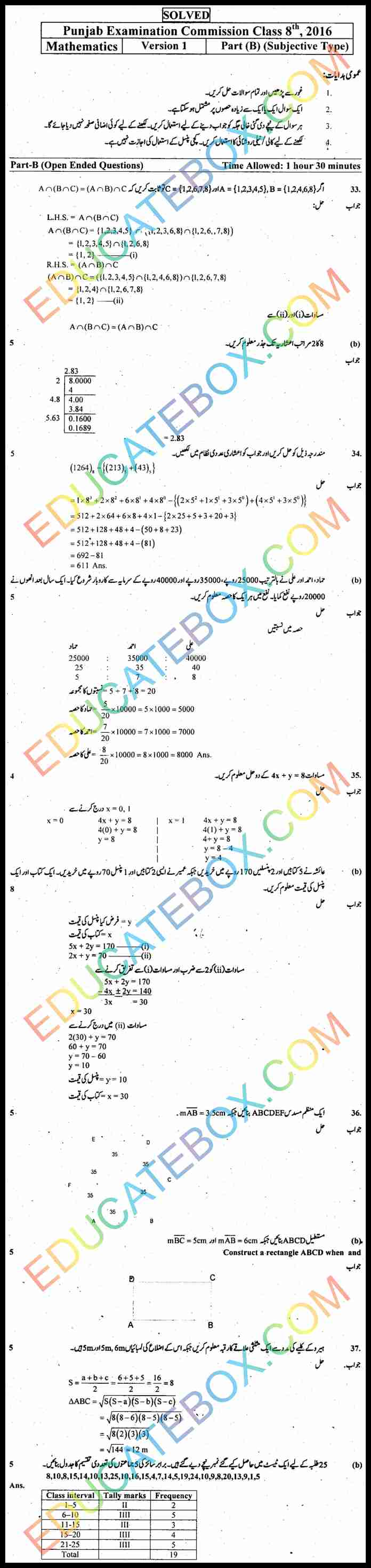# Past Paper 8th Class Maths 2016 Solved Paper UM Punjab Board (PEC) Version 1

0
1437

## Past Paper 8th Class Maths 2016 Solved Paper Punjab Board (PEC) Objective Type Urdu Medium Version 1

Punjab Examination Commission Maths paper was held on Wednesday, February 17, 2016. It is recommended that students should solve this paper by their selves then see the solution in this paper. This is for Urdu medium students.  It is a full hundred marks paper.  The first part of the paper is of 48 marks. The second part of the paper is of 52 marks. Total time allowed for the first part is one hour and fifteen minutes and time for the second part is one hour and thirty minutes.

There is a question of subset of {1,2},  another question of power set of {3,4}, rational number from a set, how many number of digits in square root of 17956, find cube of 3, a binary division question, another binary minus question, conversion question to base 5, find number of variables in a complex equation, few questions about graph, mode of data element {2, 3, 1, 4, 3, 4, 3, 5, 1}, and median of data {10, 14, 15, 17, 7, 16, 13}.

In the subjective part a question about Intersection. Calculate square of 8 up to 2 decimal places. Calculate the expression and convert the answer into decimal system. Find the solution of a complex equation.

## اپ ٹو ڈیٹ پیپر ہشتم کلاس ریاضی حل شدہ پیپر پنجاب بورڈ اوبجیکٹیو ٹائپ اردو میڈیم ورزن 1## Past Paper 8th Class Maths 2016 Solved Paper Punjab Board (PEC) Subjective Type Urdu Medium Version 1 اپ ٹو ڈیٹ پیپر ہشتم کلاس ریاضی حل شدہ پیپر پنجاب بورڈ سبجیکٹیو ٹائپ اردو میڈیم ورژن 1Save

Save